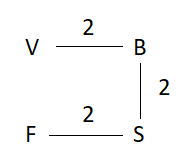Question 123

# P * Q implies that Q is 2 kms to the left of PP @ Q implies that Q is 2 kms below PP $Q implies that Q is standing 2 kms above PP ≠ Q implies that Q is standing 2 kms to the right of PIf F ≠ S$ B * V, in which direction is F with respect to V?

Solution

The given condition is as shown below:-As we can see F is to the South of V.
Hence, option B is the correct answer.

### View Video Solution

• All Quant Formulas and shortcuts PDF
• 40+ previous papers with solutions PDF